# Investigating the Multi-input Multi-output Air Conditioning Control Techniques

Investigating the Multi-input Multi-output Air Conditioning Control Techniques
Mechanical Power Department, Modern University for Technology and Information, Cairo 11431, Egypt

Nuclearand Radiological Regulatory Authority (NRRA), Cairo 11431, Egypt

Corresponding Author Email:
khaled.pasha@eng.mti.edu.eg
Page:
345-355
|
DOI:
https://doi.org/10.18280/jesa.530305
17 April 2020
|
Accepted:
31 May 2020
|
Published:
31 July 2020
| Citation

OPEN ACCESS

Abstract:

The first step of the present work is to investigate numerically many suggested control techniques for the multi-input multi-output (MIMO) control systems. A program is written to simulate the different control techniques. This program works along with a commercial code, which simulates the indoor conditions of a model room. The second step is to apply experimentally the most promising control technique to the model room which simulates a larger computer server room. In the experimental runs, it was mandatory to introduce two formulas to estimate more realistic sampling time intervals for the two controlled variables. The room is conditioned by an HVAC system which is controlled by a MIMO control system. The two controlled outputs are the temperature and humidity. The two control variables are the grill opening angle of the inlet air and the rate of humidification. This scheme was chosen because the idea of mixing the fresh and return air helps to reduce the system energy losses. Also, it is simple and less expensive. When applying accidental disturbances to both; the temperature and the humidity, the suggested technique exhibited accepted results. But, because of the narrow range of variation in the mixed air ratio, the results were not satisfying for the cases where larger permanent disturbances were present. The suggested technique is a case-independent and may be applied to any multi-input multi-output control system.

Keywords:

MIMO, control, HVAC, energy

1. Introduction

The demands for the different types of energy and its rates of consumption have greatly increased in the last few decades due to the fast-growing developments in technology and different aspects of life. Since the natural sources of energy or renewable energy are not always available to all countries, then the energy saving became the essential aim of the design process of any system that consumes energy, such as HVAC systems. The energy-saving process depends mainly on the used control technique that consists of hardware and software. One of the famous control techniques is the PID that succeeded in many applications but, it requires a suitable tuning for its parameters. Advances in the auto-tuning of the PID parameters in an adaptive manner have been developed and; both analog and digital versions exist that also include intelligent methods [1, 2]. Ghazali , modified PID gains obtained by Ziegler and Nichols by multiplying the output from each PID controller by a reducing factor. This reducing factor represents the rate of change of each control signal for each participating PID output. He simulated this modification in a 3-input/ 2-output building energy management system (BEMS) with known plant dynamics. He studied the controller sum output squared error and total energy consumption when the system is at steady-state conditions. His modified method may be extended to reduce the number of PID controllers as well as the control inputs for specified necessary outputs. The PID controllers have also widely been used in multivariable applications where their commissioning is less well formalized [1-5]. The normal approach is to use a robust de-tuning philosophy to reduce the gains so that the multivariable application can operate . Many researchers tried to achieve generic methodologies that allow PID techniques to be applied to multivariable systems and accordingly, to work with multi-loop controllers. The basics of such techniques and its tuning procedures were explained in the classical Ziegler and Nichols . Ziegler and Nichols suggested formulas that enable the controller parameters to be determined from the experimental or calculated values of the lag and unit reaction rate of the controlled process. However, most researches today prefer the simulating programs which simulate the building specifications and the thermal loads during the four seasons as well as estimating the control parameters. Although the procedure seems to be simple and convenient, it still constrained by the available program capabilities, modeling procedures, and the data stored in these simulating programs. And again, we still need to tune the controlling scheme at run time according to the actual conditions. An example of these simulation procedures is the work of Wetter , who implemented a Building Control Virtual Test Bed (BCVTB) and connected different simulation programs to exchange data during the time integration. The used software architecture is a modular design based on Ptolemy II, for the design and analysis of heterogeneous systems. He visualized the system evolution during run-time. He prepared a mathematical model to implement the co-simulation in which different simulation programs are linked for run-time data exchange to compute a solution to the coupled problem using co-simulation. Salsbury and Diamond  suggested a model-based, feed-forward control scheme to improve the control performance over traditional PID and detect faults in the controlled process. They used static simulation models of the system

Pasha  made a comparison between the PID and the other control techniques for an HVAC system. But he applied these techniques to control each one of the temperatures and humidity alone. He reported that the fuzzy technique exhibited faster convergence for both controlled variables but he did not consider the coupling effects. Anderson et al.  simulated an HVAC system and designed its controllers. They implemented their model in a true system to test different controlling methods. Their results showed that, the discharge air temperature time was reduced by 300%. Belic et al.  reviewed many control methods and concluded that, Classical PID controllers are still applied in most applications. But considering the energy, the Advanced predictive or intelligent methods exhibit better performances. Jain and Alleyne  showed that, if the correct feedback variables are chosen in the HVAC industry, the performance of a SISO control technique may approach the MIMO control technique. They performed their investigation with An LQG controller and compared their results with a decentralized controller and they exhibited a good agreement.

1.1 Objectives of the present work

The first step is to suggest and investigate many MIMO control techniques that may be applied to an HVAC control system. Next, we implemented the most promising technologies in a model room, which is conditioned by an HVAC system. In this system, both the fresh and return air are mixed at a ratio that is determined by the controller which also controls the rate of humidification. The two controlled outputs are the temperature and humidity.

This HVAC system was chosen because:

- The idea of mixing the fresh and return air helps reduce the overall consumed energy of the HVAC system.

- It could be accomplished with simple and low-cost components.

2. Numerical Simulation

2.1 Preparing the code

The real case is nonlinear and includes thermal, hydraulic and species convection and diffusion which are controlled by nonlinear differential equations. So, it is intended to simulate the transport of all conserved quantities inside the room using a commercial code, (ANSYS). We used a transient numerical scheme which is a first-order implicit and pressure-based scheme. The least-squares cell-based method is used for the gradients. A first and second-order upwind are used for both; the turbulent kinetic energy and the momentum, respectively.

To simulate the different suggested control techniques, we wrote a program that works along with the commercial code to investigate the responses of the system for each suggested control method. Appendix (A) contains the details of the written program. This program performs the following tasks:

1. Estimates the initial control signals which establish the equilibrium conditions inside the room. This step is difficult to be accomplished analytically in multi-input multi-output control systems. The equilibrium conditions were achieved with a 14.8% grill opening and a 2% humidifier opening.
2. Estimates the variation of each output with different step changes in each input.
3. Investigates the responses of the suggested control techniques.

Many suggested control formulas were simulated in addition to the classical Ziegler and Nichols method . The majority of them exhibited either, oscillating, unstable, or diverging behavior. For any multi-input multi-output control systems, each one of the inputs is most probable to affect all the outputs. Then, it is expected for any one of the outputs to achieve its set-point, but the control system continues to modify the control inputs to drive the other outputs towards their set-points too.

2.2 The proposed control technique

So, we tried to prepare a control technique that may drive all the outputs towards their set-points almost at the same time. It is explained as follows:

(1) Let the number of inputs to be “m” and the number of outputs is “n”. The first step is to simulate the variation with time in each output with different step changes in each input for a period which is enough to repair the maximum expected error in this output. The input step value is the difference between the exerted control input and the corresponding equilibrium value. From these simulations, we record the total change in every output due to each step change in every input. For our model room, we used a simulation period of 60 seconds. The used percentages of the grills opening are 5, 10, 25, 35, 45, 55, 65, 75, and 85% whereas, the used percentages of the humidifier opening are 0, 3.2, 5.2, 8.2, 10.2, 13.2, 16.2, 18.2 and 20%. Note: for “0% “humidifier opening the low moisture content in the fresh air could not maintain the humidity value inside the room and so, it kept decreasing during the process. But after increasing the humidifier opening percentage, the spread steam rate exceeded the reduction rate of the humidity.

(2) Also, the outputs changes in the first five sampling intervals are averaged for each output. This average almost represents the real-time change in the output during each sampling interval after each new modified input signal is sent to the corresponding actuator. Figure 1 illustrates the responses of the temperature, to different values of the grill opening percentages. Figure 2 illustrates the responses of the relative humidity, (represented by the moisture fraction, Eq. 17) to different values of the grill opening percentages. Figure 3 illustrates the responses of the humidity for different values of the humidifier opening percentages.

(3) The resulting data of each input with each output changes are fitted to relations in the form; uij=f(ej), (i=0, 1, …, m and j=0, 1, …, n). The value of uij is the modification of each input, ui, from its equilibrium value, ui,eq, and can change each output, (yj) by a value (ej) to return it to its set-point value, (equilibrium value, yi,eq). When using these relations to calculate the required inputs, we use the negative values of the total errors, etj,

where,

$\mathrm{e}_{\mathrm{t} \mathrm{j}}=\mathrm{e}_{\mathrm{j}}+\int_{\mathrm{ts}}^{\mathrm{tc}} \mathrm{e}_{\mathrm{j}} \mathrm{dt}$  (1)

dt” is the sampling time interval. The second term to the right-hand side of Eq. (1) started to be calculated for each error value after its sign is reversed “for the first time”, (at the sampling interval ‘ts’), and ‘tc’ is the current sampling interval. For the present work, (two inputs and two outputs), these relations were estimated to be:

$\mathrm{u}_{00}=-0.11 \mathrm{e}_{\mathrm{t} 0}-0.0132 \mathrm{e}_{\mathrm{t} 0}^{2}-0.0031 \mathrm{e}_{\mathrm{t} 0}^{3}$  (2)

$u_{01}=-1338.64 e_{t 1}-850796 e_{t 1}^{2}-5900000000 e_{t 1}^{3}$  (3)

$\mathrm{u}_{10}=0.00002 \mathrm{e}_{\mathrm{t} 0}$ (4)

$\mathrm{u}_{11}=13.97 \mathrm{e}_{\mathrm{t} 1}$ (5)

(4) The data of each output in the first five sampling intervals are averaged. These averages with their corresponding input step values are fitted to suitable relations, in the form, $\dot{y}_{i j}=f\left(u_{i j}\right)$

For the present work, these relations were estimated to be:

$\dot{y}_{00}=-0.2 \mathrm{u}_{00}-0.07 \mathrm{u}_{00}^{2}$  (6)

$\dot{y}_{10}=-u_{10} / 65430$  (7)

$\dot{y}_{01}=0.0003 u_{01}$  (8)

$\dot{y}_{11}=u_{11} / 688.3$  (9)

where, $\dot{\mathrm{y}}_{\mathrm{ij}}$ is the average change of the output yj in the first five sampling intervals due to the input ui(i=0,1,…, m, and i=0,1,…, n), Figures 1, 2, and 3.

(5) Introducing the ‘equalizing factors’, a0, a1,......, am, that could be estimated from:

$\frac{\sum_{\mathrm{i}=0}^{\mathrm{i}=\mathrm{m}} \propto_{\mathrm{j}} \dot{\mathrm{y}}_{\mathrm{ij}}}{\left|\mathrm{e}_{\mathrm{j}}\right|}=\frac{\sum_{\mathrm{i}=0}^{\mathrm{i}=\mathrm{m}} \propto_{\mathrm{j}} \dot{\mathrm{y}}_{\mathrm{ij}+1}}{\left|\mathrm{e}_{\mathrm{j}+1}\right|}, \mathrm{j}=0,1, \ldots \ldots \mathrm{n}-1$  (10)

Because ‘i’ and ‘j’ starts from the value of ‘0’, Eq. (10) represents (n) system of linear equations in $\propto_{0}, \propto_{1}, \ldots .,$ and $\propto_{m}$, with $\propto_{0}=1$

(6) Finally, after estimating the values of $\propto_{0}, \propto_{1}, \ldots .,$ and $\propto_{m}$, the formula for each input is:

$U_{i}=U_{e q, i}+\beta\left(\frac{\Sigma_{j=0}^{j=n} \alpha_{j} u_{i j}}{n}\right)$  (11)

where, ueq,i is the value of the input ui, which is required to establish the equilibrium conditions for all controlled variables. As mentioned before, uijis calculated for the error etj. The potency factor, $\beta$ represents compensation to the lack of any input potency to transfer its effect to the controlled variables. It differs from case to case, and in the present work, as an example, it may be estimated as follows:

$\beta_{\mathrm{T}}=| \frac{\mathrm{T}_{\mathrm{r}, \mathrm{l}}-\mathrm{T}_{\mathrm{f}}}{\mathrm{T}_{\mathrm{r}}-\mathrm{T}_{\mathrm{f}}}|$  (12)

$\beta_{\mathrm{m}}=| \frac{\omega_{\mathrm{r}, \mathrm{l}}-\omega_{\mathrm{f}}}{\omega_{\mathrm{r}}-\omega_{\mathrm{f}}}|$  (13)

Tr,1 and wr,1 are the largest recorded temperature and humidity values, respectively, during the whole time.

## 1a.jpg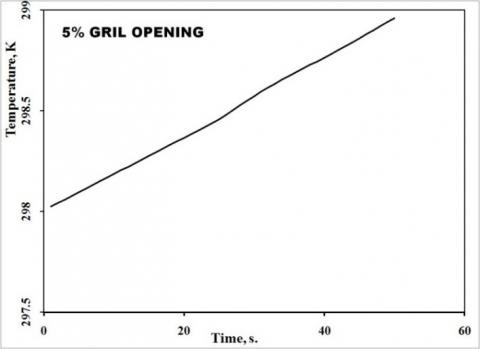## 1b.jpg## 1c.jpg## 1d.jpgFigure 1. The responses of the temperature for some values of the grill opening percentages

## 2a.jpg## 2b.jpg## 2c.jpg## 2d.jpgFigure 2. The responses of the humidity for some values of the grill opening percentages

## 3a.jpg## 3b.jpg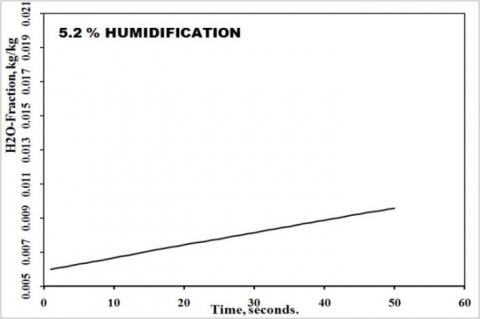## 3c.jpg## 3d.jpg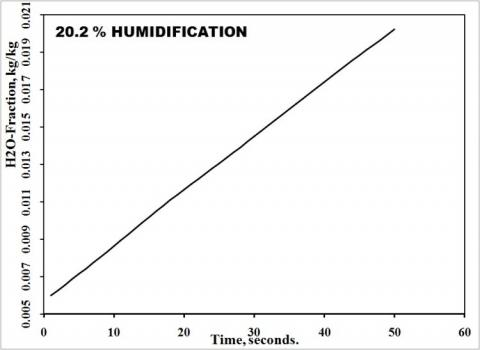Figure 3. The variations of the humidity for some values of the humidifier opening percentages

3. Experimental Preparations

The test room, Figure 4 (a, b and c), has a length, width, and height of 3, 3, and 2 m, respectively, and its wall is composed of two layers; gypsum plaster, [k=0.17 W/m·oC] and asbestos insulation [k=0.2W/m·oC]. Each one of these layers has a thickness of 1.5 inches. two circular ports are located on the left side wall, each one has a diameter of 30 cm. The center of each port is at height 170 cm from the floor. One of the ports is for the inlet air mixture and the other is for the return air. The test room is constructed to simulate a real room whose length, width, and height are 6, 6, and 4 m respectively. This room contains two main servers with their accessories. The indoor temperature and relative humidity should not exceed 25oC and 30% respectively. The thermal loads, the maximum inlet air rate, and the maximum humidifying steam rate were scaled according to the volume ratio of the test and real rooms. The electronic types of equipment are simulated by two blocks; each one is made of steel sheets, whose length, width and height are 1, 0.5, and 1 m, respectively. Each block contains a 0.3 kW heater, so the heat flux is 86 W.m-2. In the center of the ceiling, a 0.2 kW lamp was fixed to simulate the room light. The lamp area is 0.07 m2 and accordingly, the heat flux is 2828 W.m-2. To produce the disturbances, the room is equipped with auxiliary heater and humidifier that will be operated for a certain period until the temperature is 28oC and the relative humidity is 29, which corresponds to absolute humidity of 6.5 g moisture/kg dry air.

The air handling unit is a well-isolated mixing room whose length, width, and height are 1.5, 1.0, and 0.6, respectively. It contains two air openings for the return and mixed air, each one has a diameter of 0.3 m. Each opening is followed by a filter and a movable grill. It contains also a suction fan which is driven by an AC induction motor. A cooling coil is located in the passage of the fresh air. It works as a heat exchanger that receives the chilled water from a refrigeration unit and cools the inlet fresh air

## 4a.jpg(a) photograph

## 4b.jpg(b) the control system details

## 4c.jpg(c) the used grid to simulate the model room

Figure 4. The model room

The chiller unit consists of one HP refrigeration unit that works with Freon, (R-23). Its compressor is operated by a variable speed motor to control the cooling capacity, and accordingly, the inlet fresh air temperature. The refrigerant is chilling the water, which discharges from a half-HP water pump. This chilled water enters the cooling coil in the air handling unit, through a half-inches insulated pipes, where, it cools the fresh air.

The Control system consists of an 8051 Architecture Microcontrollers which is a rich Atmel portfolio of MCUs based on the 8051 instruction set. This microcontroller was connected to the computer and receive the temperature and R.H readings from five DHT11 sensors. Four of these sensors are settled along the way from the mixed air inlet to the farthest position in the room from the air inlet. The fifth sensor is located in the entrances of the cooled fresh air. The readings from this sensor tell the controller whether it must increase or decrease the compressor speed to keep the fresh air within certain temperature limits. These temperature data will be used in the numerical simulations. The controller sends control signals to three stepper motors through Ease Driver V4.5 A3967. These motors control the openings of the fresh air, the return air, and the humidifier. At any moment, the grills of each opening are inclined at the same angle due to the gearing system, Figure 5. To ensure almost a fixed rate of inlet air mixture during any experiment, the fresh and return air openings must always be maintained in positions such that, the sum of their opening area equals the total entrance area. The humidifier, Figure 6 consists of a container that is filled with pure water. Also, a pressure regulator maintains a suitable spraying pressure at the nozzle.

## 5.jpgFigure 5. The inlet port for either

## 6.jpg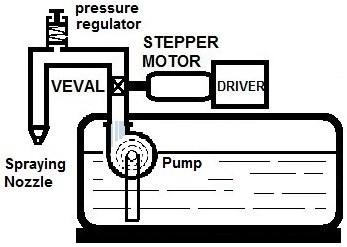Figure 6. The humidifier conditioned or return air

In the experimental application, the controller keeps calculating the ratios of the fresh and return air to the total air mixture flow rate. These ratios must be translated into angle ratios of the grill’s rotation angle to the full opening angle. These angle ratios are converted into analog signals that will be fed to the stepper motors of the grills. Returning to Figure 5, the relations between the air mass ratios and the corresponding changes in the air opening angles, (dqf and dqr) are derived as follows:

$\left(\dot{\mathrm{m}}_{\mathrm{f}} / \dot{\mathrm{m}}_{\mathrm{t}}\right)_{new}=\left(\mathrm{A}_{\text {open }} / \mathrm{A}_{\text {total }}\right) _{f,new}=1-\cos \left(\theta_{\text {previous }}+\right.$$\mathrm{d} \theta)_{\mathrm{f}} (14) From Eq. (14), (dq f) is estimated and \left(\mathrm{A}_{\text {open }} / \mathrm{A}_{\text {total }}\right)_{\mathrm{r}, \text { new }}=1-\left(\mathrm{A}_{\text {open }} / \mathrm{A}_{\text {total }}\right) _{f}=1-$$\cos \left(\theta_{\text {previous }}+\mathrm{d} \theta\right)_{\mathrm{r}}$  (15)

From Eq. (15), (dq r) could be determined from:

$\mathrm{d} \theta_{\mathrm{r}}=1.6-0.41 \cos (\theta)_{\mathrm{r}}-3.54 \cos (\theta) \mathrm{r}^{2}+6.68$$\cos (\theta) r^{3}-4.2 \cos (\theta) r^{4}-\left(\theta_{\text {previous }}\right)_r$  (16)

The other control variable, (the humidity) is controlled by another analog signal which represents the ratio of the change in the valve opening angle to the full opening angle. That is because the relation between the angle of rotation and the steam flow rate is linear.

The current temperature and the relative humidity are the averages of those values at four specified positions, Figure 4b.

The moisture fraction in the air could be estimated from the corresponding measured temperature and the relative humidity as follows:

$m_{f}=\frac{\omega}{1+\omega}$  (17)

And the absolute humidity is calculated from the measured temperature and relative humidity using:

$\omega=\frac{-0.622 \emptyset \emptyset\left(-1538+16.7 \mathrm{T}-0.06 \mathrm{T}^{2}\right)}{\emptyset \emptyset\left(-1538+16.7 \mathrm{T}-0.06 \mathrm{T}^{2}\right)-101.3}$                             (18)

4. Results and Discussion

4.1 Numerical simulations

In the present work, many suggested formulas to control an HVAC system in a model room were simulated. The two outputs are temperature and humidity. The two control inputs are the grill opening angle and the steam flow rate from the humidifier. The permanent disturbance in the temperature was simulated numerically by increasing the load's heat flux, whereas, the permanent disturbance in the humidity was simulated by adding a fixed source of moisture. The majority of the investigated control methods exhibited either, oscillating, unstable, or diverging behavior. Figure 7 illustrates the output responses in two of these cases, whose formulas are:

$u_{i}=u_{e q, i}+r_{-} e \Delta u_{i} / \alpha_{e_{i}}+I_{i} / \alpha_{I . i}$  (19)

$\mathrm{u}_{\mathrm{i}}=\mathrm{u}_{\mathrm{eqi}}+\Delta \mathrm{u}_{\mathrm{t}, \mathrm{i}}$  (20)

ui=input signal, ueq,i=equilibrium input signal and $\Delta \mathrm{u}_{\mathrm{i}}$=change in input signal from the value of ueq,i.

$\Delta u_{t, i}=\frac{\sum_{j=0}^{j=n} u_{i j}}{n}$  (21)

where, Ii is the integral of the error (i) and uave,i is the average input, (i).

$\dot{\mathrm{y}}_{\mathrm{ij}}$ are the rates of changes of outputs (j) due to inputs (i). They are estimated experimentally and then, fitted to uij, Eqns. (6-9). Next, they are modified by the factors $\alpha_{\mathrm{ei}}$.

To get $\alpha_{\mathrm{e} 0}$, as example, we apply Eq.  to $\dot{\mathrm{y}}_{00}, \dot{\mathrm{y}}_{01}, \dot{\mathrm{y}}_{10}, \dot{\mathrm{y}}_{11}, \mathrm{e}_{0}$ and $\mathrm{e}_{01}$, to get $\alpha_{\mathrm{e} 0},\left(\dot{\mathrm{y}}_{11} /\left|\mathrm{e}_{1}\right|-\dot{\mathrm{y}}_{10} /\left|\mathrm{e}_{0}\right|\right) /\left(\dot{\mathrm{y}}_{00} /\left|\mathrm{e}_{0}\right|-\dot{\mathrm{y}}_{01} /\left|\mathrm{e}_{1}\right|\right)$.

uij,o is calculated from ej Eqns. (2-5).

$\mathrm{r}_{-} \mathrm{e}=\left|\mathrm{e}_{0}+\mathrm{e}_{1}\right| /\left|\mathrm{Le}_{0}+\mathrm{Le}_{1}\right|$  (22)

Lei = largest value of each error, ei during the whole control process.

The reason for these results may be interpreted as follows, in the present investigation, and many other applications, every input affects all outputs. So, we suggested two techniques, and we think that each one may deal with these conditions and drives the outputs to their set-point values. When simulating the suggested technique, it exhibited promising results with the accidental disturbances in the temperature and humidity. Also, the control scheme of air mixing offers a limited range of input variation, and the controller, in some times during the process, works as an ON\OFF controller.

## 7a.jpg## 7b.jpg## 7c.jpg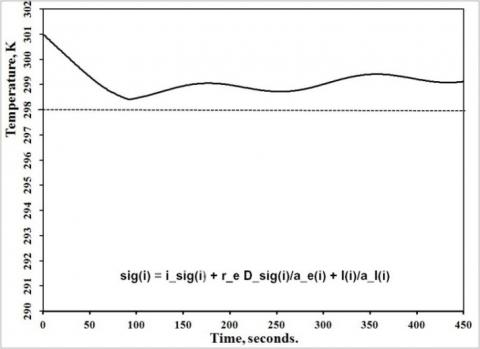## 7d.jpgFigure 7. The temperature and the humidity responses for two of the investigated control formulas

Figure 8 illustrates the variation in the temperature and the humidity, when applying accidental disturbances in both; the temperature and the humidity. The figure illustrates that both outputs attained their set-point values on the time between 250 and 300 seconds but, the diffusion of both the thermal energy and the moisture continued to decrease the two outputs. So, although the controller reversed the signs of both signals, there were delays in the outputs responses. As mentioned in the technique description, the integral of each output errors start to be calculated after its value approaches the zero value, Eq. (1), and this supports the damping process for both outputs changes after approaching their set-point values. But, when applying it to the case of accidental with permanent disturbances, the results were not satisfying.

Figure 9 illustrates the variation in the temperature and the humidity when applying an accidental with large permanent disturbance in both outputs. That is because the accidental and permanent disturbances together produce larger error values. The accuracies of Eqns. (2-5) were found to deteriorate when these equations are applied to these larger values. Moreover, the two inputs have opposing effects on the humidity of, so, for large permanent disturbance in one output will lead to unstable variation in the other output. All these conditions will lead to misleading calculated input values that are not capable of driving the outputs correctly. So, the control variables have narrower ranges of variation when controlling the humidity, and accordingly, when applying smaller permanent disturbance in the humidity the controllers exhibited better behavior. Figure 10 illustrates the responses of the temperature and the humidity after accidental disturbances with a smaller permanent disturbance in the humidity. (simulation of the suggested technique).

## 8a.jpg## 8b.jpgFigure 8. The responses of the temperature and the humidity after accidental disturbances (simulation of the suggested technique)

## 9a.jpg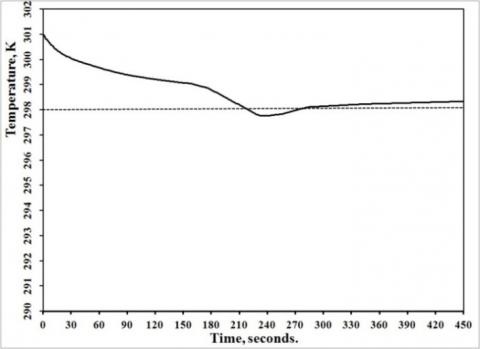## 9b.jpg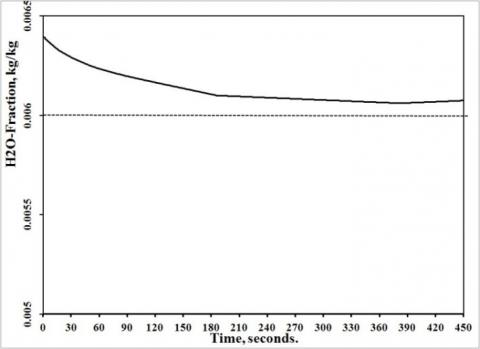Figure 9. The responses of the temperature and the humidity after (accidental and large permanent) disturbances (simulation of the suggested technique)

## 10a.jpg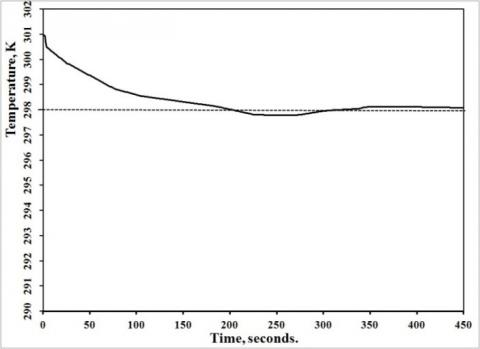## 10b.jpgFigure 10. The responses of the temperature and the humidity after accidental disturbances with a smaller permanent disturbance in the humidity (simulation of the suggested technique)

4.2 Experimental results

When applying the suggested control technique to the model room in the real-time test, the variations in both the temperature and humidity were not convergent. In some tests, the variations in the two controlled variables were unstable. In other cases, these variations were oscillating. Besides the unpredicted differences between the real-time and the simulation tests, there is an important factor that has a great influence on the control process, and this factor is the sampling time interval. In the numerical simulation, the temperature and humidity are calculated after any chosen time interval. But in the real-time test, the temperature and humidity are measured by the probes. The sensitivities of these probes determine the limits of the sensed changes in the measured variables. $\Delta \mathrm{T}$ and $\Delta \emptyset$ that could be converted into $\Delta \omega$ using Eqns. (17 and 18). So, any changes that are smaller than the sensed values will result in misleading readings and great errors in the calculations that are based on these readings. Each sampling time interval must be larger than the time which is required for the measured variable to change by the sensed values of the used probes. To estimate approximate values for these sampling time intervals, we used simple energy balance for the whole system as follows:

$\tau_{\mathrm{T}}=\frac{\rho V\left[\left(C_{\mathrm{P}}+1.82 \omega_{\mathrm{av}, \mathrm{in}}\right) \Delta \mathrm{T}\right]}{\mathrm{l}_{\mathrm{T}}+\mathrm{m}_{\mathrm{m}}\left(\mathrm{C}_{\mathrm{P}}+1.82 \omega_{\mathrm{av}, \mathrm{mix}}\right)\left(\mathrm{T}_{\mathrm{r}}-\mathrm{T}_{\mathrm{m}}\right)}$  (23)

$\operatorname{l}_{\mathrm{m}}=\frac{>\mathrm{V} \Delta \omega]}{\mathrm{l}_{\mathrm{m}}+\mathrm{m}_{\mathrm{m}}\left[\left(\omega_{\mathrm{r}}+\frac{\Delta \omega}{2}\right)-\left(\omega_{\mathrm{m}}+\frac{\Delta \omega}{4}\right)\right]}$  (24)

During the time $\tau_{\mathrm{m}}, \omega_{\mathrm{r}}$ will change by a value $\Delta \omega$, and its average value is $\omega_{r}+\Delta \omega / 2$. Accordingly, the value of $\omega_{\mathrm{m}}$ may be approximated to $\omega_{\mathrm{m}}+\Delta \omega / 4$.

$\omega_{\mathrm{av}, \mathrm{mix}}$ is the average of the two absolute humidity values that are measured for the fresh and return air.

$\omega_{\mathrm{av}, \mathrm{in}}$, is the average of the two absolute humidity values that are measured for the indoor air at the current temperature and at the temperature that is incremented by $\Delta \mathrm{T}$ .

The pressure inside the room is almost atmospheric, so, the air density, (r=Pa/RT) may be calculated for each sampled temperature.

The program uses these calculated time-intervals as the sampling time intervals. Figure 11 illustrates the experimental results of the variation in the temperature and the humidity when applying the suggested technique with accidental disturbances in both outputs. The two outputs approached their set-point values within a range of time between 200 and 230 seconds which is the main goal of the suggested technique. After approaching their set-point values, the smaller errors in some output will have smaller effects on the other outputs, and that supports the stability of the variations of the output until achieving their set-point values.

4.3 A comparison with some previous work

To check the reliability of the suggested technique we compared the behavior of our suggested control technique with that of a published case that is very close to our controlled system. In this case, the HVAC system is controlled by a fuzzy technique. Figure 12 illustrates the behaviors of the published fuzzy technique and our suggested technique. From the figure, it is obvious that, using a fuzzy technique in Khaled’s work drove the temperature towards the setpoint value faster than that in the present work. But, the fuzzy technique in this paper controlled each one of the controlled variables alone regardless of the behavior of the other controlled variables. In addition, the oscillation amplitudes of the controlled variable after approaching the setpoint decreased considerably in the present work.

## 11a.jpg## 11b.jpgFigure 11. The responses of the temperature and the humidity with “accidental” disturbances in both outputs. Experimental results of the suggested control technique

## 12.jpg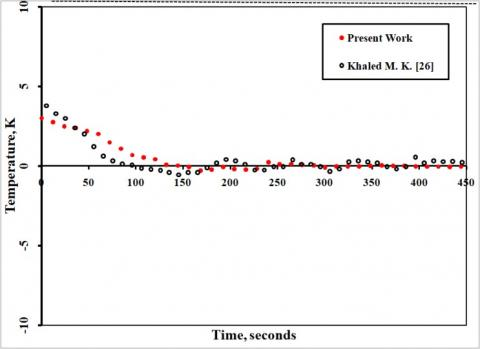Figure 12. The temperature responses to merging disturbances for the present work and Khaled .

5. Conclusion

In the present work, we are looking for a control technique that is suitable for the cases of multi-input multi-output control systems.

The investigation is performed for the case of two inputs-two outputs HVAC control system in a computer server room.

The two control inputs are the opening angle of a fresh air gate and the steam flow rate from a humidifier, and the two controlled outputs are the temperature and the humidity.

This control system is chosen because it is simple and less expensive. It helps reduce system energy losses.

A written code along with a commercial code was used to simulate both; the indoor conditions and the HVAC control process inside a model room which simulates a real computer server room.

Between all investigated techniques, one of them exhibited accepted results for cases of accidental disturbances. But when applying accidental disturbances with larger permanent disturbances, the results were not satisfying.

This simple HVAC control scheme is suitable for applications where small permanent disturbances are expected.

The suggested technique is case-independent and could be applied to any multi-input multi-output control system.

Nomenclature

Alphabetic

 A area, m2 CP specific heat of air at constant pressure, kJ/kg-1. K-1 e error of one controlled variable h enthalpy, kJ.kg- lT thermal loads, W lm sources of moisture, kg. s-1 L grill length, m $\dot{\mathrm{m}}$ mass flow rate, kg. s-1 M signal from one controller to one actuator mf moisture fraction, kg/kg taw response lag, sec. T temperature, K. U total control signal to one actuator Y controlled variable Greek symbols $\alpha_{i}$ equalizing factor for input ui $\beta_{\mathrm{T}}$ potency factor for the temperature $\beta_{\omega}$ potency factor for the humidity $\Delta \mathrm{T}$ the smallest temperature change that could be sensed by the probe, K $\Delta \omega$ the smallest humidity change that could be sensed by the probe, kg/kg $\phi$ the relative humidity $\theta$ the grill inclination angle, rad. $\tau_{\mathrm{T}}$ minimum sampling periods for the temperature, sec. $\tau_{\mathrm{m}}$ minimum sampling periods for the relative humidity, sec. $\omega$ humidity Subscripts a air eq equilibrium conditions f i, j m r s sk t fresh (conditioned) air indices mixture, (fresh and return air) return air steam condition of smallest output summation total Abbreviations HVAC Heating Ventilation and Air Conditioning MIMO PID RH multi-input multi-output proportional, integral and differential relative humidity
References

 Savran, A. (2013). A multivariable predictive fuzzy PID control system. Applied Soft Computing, 13(5): 2658-2667. http://dx.doi.org/10.1016/j.asoc.2012.11.021

 Chang, W.D. (2007). A multi-crossover genetic approach to multivariable PID controllers tuning. Expert Systems with Applications, 33(3): 620-626. http://dx.doi.org/10.1016/j.eswa.2006.06.003

 Ghazali, A.B. (2015). A PID de-tuned method for multivariable systems, applied for HVAC plant. IOP Conf. Series: Materials Science and Engineering, 88(1): 012058. http://dx.doi.org/10.1088/1757-899X/88/1/012058

 Dittmar, R., Gill, S., Singh, H., Darby, M. (2012). Robust optimization-based multi-loop PID controller tuning: A new tool and its industrial application. Control Engineering Practice, 20(4): 355-370. http://dx.doi.org/10.1016/j.conengprac.2011.10.011

 Loh, A.P., Hang, C.C., Quek, K., Vasnani, U. (1993). Autotuning of multiloop proportional-integral controllers using relay feedback. Ind. Eng. Chem. Res., 32(6): 1102-1107. https://doi.org/10.1021/ie00018a017

 Ziegler, J.G., Nichols, N.B. (1942). Optimum settings for automatic controllers. ASME Trans., 64: 759-768. https://doi.org/10.1115/1.2899060

 Wetter, M. (2011). Co-simulation of building energy and control systems with the building controls virtual test bed. Journal of Building Performance Simulation, 4(3): 185-203. http://dx.doi.org/10.1080/19401493.2010.518631

 Salsbury, T.I., Diamond, R.C. (2001). Fault detection in HVAC systems using model-based feed-forward control. Energy and Buildings, 33(4): 403-415. https://doi.org/10.1016/S0378-7788(00)00122-5

 Blasco, C., Monreal, J., Ben´ıtez, I., Lluna, A. (2012). Modelling and PID Control of HVAC System According to Energy Efﬁciency and Comfort Criteria. In: M’Sirdi N., Namaane A., Howlett R.J., Jain L.C. (eds) Sustainability in Energy and Buildings. Smart Innovation, Systems and Technologies, vol 12. Springer, Berlin, Heidelberg. https://doi.org/10.1007/978-3-642-27509-8_31

 Homod, R.Z., Sahari, K.S.M., Mohamed, H.A.F., Nagi, F. (2010). Hybrid PID-cascade control for HVAC system. International Journal of Systems Control, 1(4): 170-175.

 Wang, Y.G., Shi, Z.G., Cai, W.J. (2001). PID autotuner and its application in HVAC systems. Proceedings of the American Control Conference, Arlington, VA June 25-27, 2001, pp. 2192-2196.

 Hossein, M., Sadati, H., Maryam, G., Hamid, T. (2008). Control techniques in heating, ventilating, and air conditioning (HVAC) systems. Journal of Computer Science, 4(9): 777-783. https://doi.org/10.3844/jcssp.2008.777.783

 Wang, J.J., Jing, Y.Y., An, D.W. (2006). Study of neuron adaptive PID controller in a single-zone HVAC system. Proceedings of the First International Conference on Innovative Computing, Information and Control, 2: 142-145. https://doi.org/10.1109/ICICIC.2006.352

 Ogonowski, S. (2010). Modeling of the heating system in a small building for control. Energy and Buildings, 42(9): 1510-1516. https://doi.org/10.1016/j.enbuild.2010.03.021

 Pasha, K. (2011). Comparison of fuzzy and (PID) techniques in controlling an HVAC system. Journal of American Science, 7(12): 475-482.

 Anderson, M., Buehner, M., Young, P., Hittle, D. (2008). MIMO robust control for HVAC systems. IEEE Transactions on Control Systems Technology, 16(3): 475-483. https://doi.org/10.1109/TCST.2007.903392

 Belic, F., Hocenski, Z., Sliskovic, D. (2015). HVAC control methods - A review. 19th International Conference on System Theory, Control and Computing (ICSTCC), Cheile Gradistei, Romania. https://doi.org/10.1109/ICSTCC.2015.7321372

 Jain, N., Alleyne, A.G. (2009). Comparison of SISO and MIMO control techniques for a diagonally dominant vapor compression system. 2009 American Control Conference Hyatt Regency Riverfront, St. Louis, MO, USA, pp. 1580-1585. https://doi.org/10.1109/ACC.2009.5160155